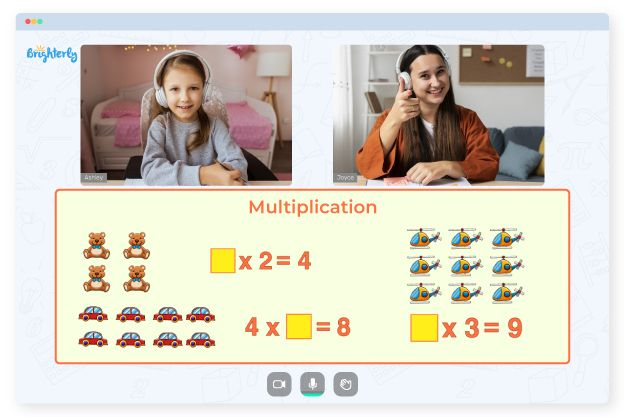# 3 Digit Multiplication Worksheets

Introducing a three digits multiplication worksheet to kids helps them get familiar with different types of multiplication problems with 3-digit numbers. The tasks could appear as word problems, multiple-choice questions, equations, etc.

Three digit multiplication worksheet is an essential concept of mathematics that allows kids to lay a mathematical foundation right from the basic math to the advanced level. These math worksheets can help young students strengthen the knowledge of the concept, gain confidence and multiplication skills, as well as challenge them to solve even more complex problems.

## Benefits of a Multiply 3 Digit Numbers Worksheet

Multiplying a three-digit number by other numbers requires memorizing multiplication tables and a basic understanding of the multiplication operation. Reinforcing this knowledge through practice enables kids to easily attain conceptual fluency for performing three-digit multiplication. Triple digit multiplication worksheets help youngsters master the skills of operating with large numbers to quickly and easily solve multiplication problems.

Math for Kids

Is Your Child Struggling With Math?
1:1 Online Math TutoringA multiplying 3 numbers worksheet comprises several exercises and multiplication problems with 3-digit numbers to encourage effective practice in kids. These three-digit multiplication worksheets contain various questions to improve a child’s conceptual fluency. The exercises are well-structured, sequentially allowing students to gain knowledge of complex concepts step by step.### 3 Digit Multiplication Worksheets PDF

3 Digit By 3 Digit Multiplication Worksheets PDF### 3 Digit Multiplication Worksheets PDF

Triple Digit Multiplication Worksheets### 3 Digit Multiplication Worksheets PDF

Three Digits Multiplication Worksheets### 3 Digit Multiplication Worksheets PDF

Three Digit Multiplication Worksheet

By downloading 3 digit by 3 digit multiplication worksheets PDF and practicing, children can understand the basics of three-digit multiplication and learn to easily solve related word problems.

### More Multiplication Worksheets

Trouble with Multiplication?• Is your child having difficulties with multiplication?
• An online tutor could be the solution.

Does your child need additional help with multiplication concepts? Try learning with an online tutor.